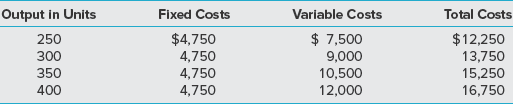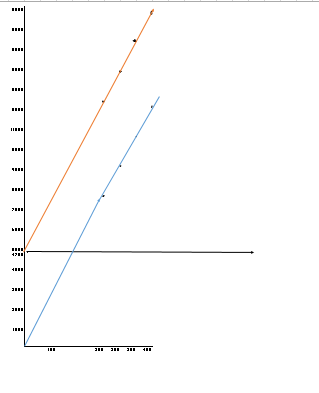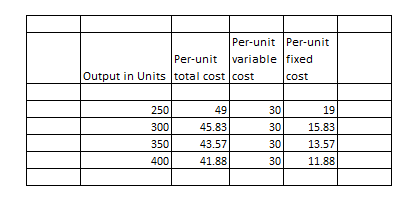# Output in UnitsFixed CostsVariable CostsTotal Costs\$ 7,5009,00010,50012,000\$4,750\$12,25013,75015,25016,7502503004,7503504,7504,750400

Question
50 views

Cost Relationships The following costs are for Optical View Inc., a contact lens manufacturer:

Required

1. Calculate and graph total costs, the total variable cost, and total fixed cost.

2. For each level of output calculate the per-unit total cost, per-unit variable cost, and per-unit fixed cost.

3. Using the results from requirement 2, graph the per-unit total cost, per-unit variable cost, and per-unit fixed cost, and discuss the behavior of the per-unit costs over the given output levels.help_outlineImage TranscriptioncloseOutput in Units Fixed Costs Variable Costs Total Costs \$ 7,500 9,000 10,500 12,000 \$4,750 \$12,250 13,750 15,250 16,750 250 300 4,750 350 4,750 4,750 400 fullscreen
check_circle

Step 1

Total Cost of production includes both fixed cost and variable costs. Variable costs are those which vary with each level of output and fixed costs remain fixed over a period of time and are independent of the production level.

Step 2The units produced have been plotted on x axis and costs on the y-axis.The blue line denotes the variable cost curve, black line, fixed cost and red total fixed costs.

Step 3

2....

### Want to see the full answer?

See Solution

#### Want to see this answer and more?

Solutions are written by subject experts who are available 24/7. Questions are typically answered within 1 hour.*

See Solution
*Response times may vary by subject and question.
Tagged in